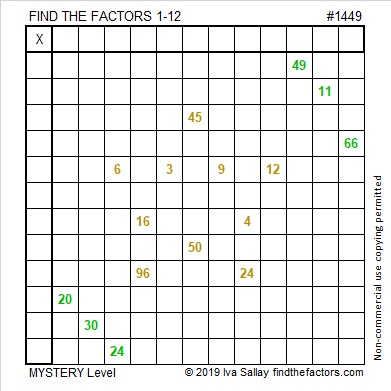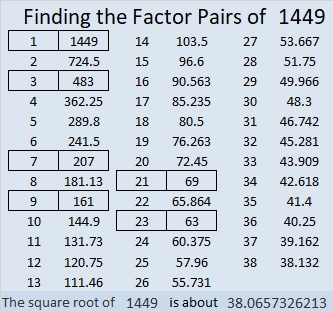# 1449 Christmas Star

If you’ve ever wished you knew the multiplication table better, then make that wish upon this Christmas star. If you use logic and don’t give up,  then you can watch your wish come true!I number the puzzles to distinguish them from one another. That star puzzle is way too big for a factor tree made with its puzzle number:Here’s more about the number 1449:

• Prime factorization: 1449 = 3 × 3 × 7 × 23, which can be written 1449 = 3² × 7 × 23
• 1449 has at least one exponent greater than 1 in its prime factorization so √1449 can be simplified. Taking the factor pair from the factor pair table below with the largest square number factor, we get √1449 = (√9)(√161) = 3√161
• The exponents in the prime factorization are 2, 1, and 1. Adding one to each exponent and multiplying we get (2 + 1)(1 + 1)(1 + 1) = 3 × 2 × 2 = 12. Therefore 1449 has exactly 12 factors.
• The factors of 1449 are outlined with their factor pair partners in the graphic below.1449 is the difference of two squares in 6 different ways:
725² – 724² = 1449
243² – 240² = 1449
107²-100² = 1449
85² – 76² = 1449
45² – 24² = 1449
43² – 20² = 1449

## 3 thoughts on “1449 Christmas Star”

1.suevanhattum

I printed this puzzle and tried it. I messed up and printed it again. Now I’m thinking it’s impossible. I may be making a mistake…

(I think I could prove that) The 5th row has to be 3s. That makes the 3rd column 2.

(I think I could prove that) The 4th column has to be 8s. (96 is 12*8 and no other factoring with both factors 12 or less. 16 is not a multiple of 12.)

That makes the 7th row 2s. But that makes the 8th column 2s and we already had 3rd column is 2s. I believe I have a contradiction. Am I wrong?

•ivasallay

The 5th row has to be either 3 or 1. Don’t start there. Start with the column that has 16 and 96 instead.

2.suevanhattum

Thank you. I was afraid I was missing something… I’ll try again. (I was going to use these for (college) students who don’t have their multiplication facts down. They may be too hard for that. But they might help teach logic. I’ll be teaching discrete math in the coming semester, and this on might be good for that.)

This site uses Akismet to reduce spam. Learn how your comment data is processed.